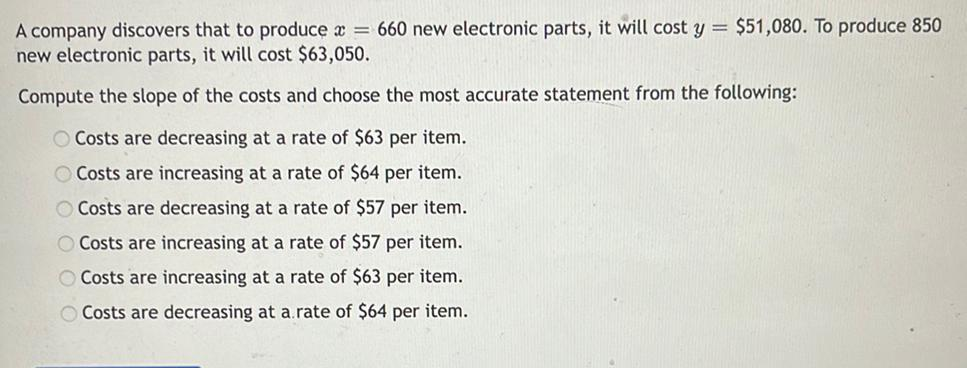Question:

# A company discovers that to produce x = 660 new electronic parts, it will cost y = \$51,080. To produce 850 new electronic parts, it will cost \$63,050. Compute the slope of the costs and choose the mosA company discovers that to produce x = 660 new electronic parts, it will cost y = \$51,080. To produce 850 new electronic parts, it will cost \$63,050. Compute the slope of the costs and choose the most accurate statement from the following: ○ Costs are decreasing at a rate of \$63 per item. ○ Costs are increasing at a rate of \$64 per item. ○ Costs are decreasing at a rate of \$57 per item. ○ Costs are increasing at a rate of \$57 per item. ○ Costs are increasing at a rate of \$63 per item. ○ Costs are decreasing at a rate of \$64 per item.